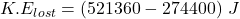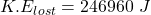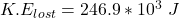## 1. On the planet Microid, trains that run on air tracks much like the air track you used in lab are the principle means of transportation. A

Question

1. On the planet Microid, trains that run on air tracks much like the air track you used in lab are the principle means of transportation. A train car with a mass of 6300 kg is traveling at 12.0 m/s when it strikes a second car moving in the same direction at 2.2 m/s. The two stick together and move off with a speed of 4.00 m/s. What is the mass of the second car? Explain your reasoning. (10 pts) 2. How much kinetic energy was lost in the collision? (10 pts)

in progress 0
2 months 2021-07-29T10:24:46+00:00 1 Answers 2 views 0

a) the mass of the second car = 28000 kg

b) the amount of kinetic energy that was lost in the collision =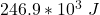Explanation:

Given that:

mass of the train car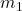= 6300 kg

speed of the train car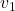= 12.0 m/s

mass of the second moving car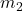= ???

speed of the second moving car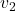= 2.2 m/s

After strike;

they both move with a speed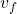= 4.00 m/s

a)

Using the conservation of momentum :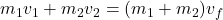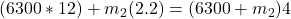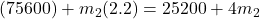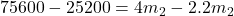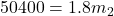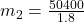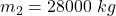b)

To determine the amount of kinetic energy that was lost in the collision;

we will need to find the difference between the kinetic energy before the collision and after the collision;

i.e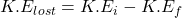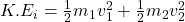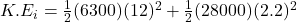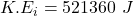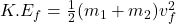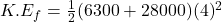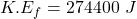Now;  the  kinetic energy that was lost in the collision is calculated as follows: